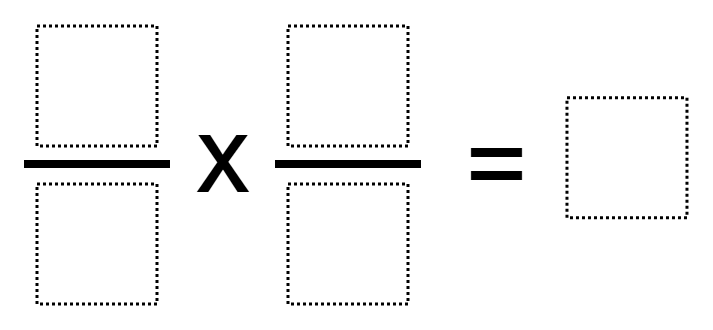# Multiplying Fractions to Make a Whole Number

Directions: Using the digits 1 to 9, at most one time each, place a digit in each box to make a whole number product.### Hint

How can you use equivalent fractions to find the answer?

6/2 * 1/3 = 1, 8/4 * 1/2 = 1, 2/4 * 6/3 = 1

Source: Owen Kaplinsky

## Add Fractions with Decimal Sums

Directions: Using the digits 1 to 9 at most one time each, place a digit …

1.My students came up with more solutions:

2/1 X 9/6 = 3
4/1 X 6/3 = 8
6/4 X 2/3 = 1
3/2 X 6/9 = 1
4/2 X 3/1 = 6
3/6 X 4/2 = 1
2/3 X 9/6 = 1
4/8 X 6/3 = 1
9/3 X 4/2 = 6

2.I did 2/1 times 9/6=3

3.4.I had 6/2 x 3/1 = 9. I got this by finding a number that times by something would be under ten. I first got 6/2 and that got me 3. Then i got 3 from 3/1. Lastly i multiplied 3 by 3 and got 9. I agree it was a challenging puzzle.

5.I got 8/1 x 6/2 = 21

6.This is not what I was looking for please be more accurate with the answers I really HATE it when I get the answers wrong. 🙁

7.2/3×1/3=3/3 or 1

•2/3 x 1/3 is 2/9, not 1.

8.I don’t agree with the first two provided answers. If you use a unit fraction as a factor, you cannot reuse the “1” tile in the answer. In fact, I don’t think there is an answer with a unit fraction as a factor. The third supplied answer works, though.

9.I got 8/6 x 3/4=1. Im 10! This is not really 5th grade level if you know how to multiply fractions.

10.Vanessa Churchill

Today we found:

9/6 x 2/3 = 1
6/9 x 3/2 = 1
6/3 x 2/4 = 1
6/8 x 4/3 = 1
4/6 x 3/2 = 1
1/8 x 4/3 = 6
6/4 x 8/3 = 2